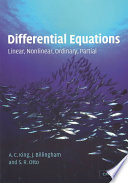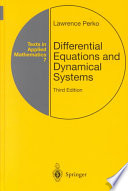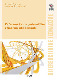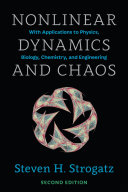### Differential Equations and Dynamical Systems

#### Course Description

Legendre, Chebyshev and Hermit functions and differential equations. Qualitative analysis of differential equations presented on the model of Euler and Bessel differential equations. Oscillations and method of Riccati differential equation. Explicit, numerical and asymptotic methods for differential equations and systems. Models and applications: oscillators, pendulums, nonlinear electronic circuits. Robustness of discrete and continuous systems, Lyapunov stability and bifurcations. Saddle-node bifurcation, Hopf, Hopf-Takens, Bogdanov-Takens bifurcations. Chaos and criteria for chaos in systems. Lorenz system.

#### LiteratureA. C. King, J. Billingham, S. R. Otto (2003.), Differential Equations, Cambridge University PressLawrence Perko (2008.), Differential Equations and Dynamical Systems, Springer Science & Business MediaLuka Korkut, Vesna Županović (2009.), Diferencijalne jednadžbe i teorije stabilnosti, Element, ZagrebSteven H. Strogatz (2014.), Nonlinear Dynamics and Chaos, Westview Press
Mervan Pašić (2013.), Fite-Wintner-Leighton type oscillation criteria for second-order differential equations with nonlinear damping, Abstract and Applied Analysis
Mervan Pašić (2007.), Rectifiable and unrectifiable oscillations for a class of second-order linear differential equations of Euler type, Journal of Mathematical Analysis and Application

#### General

ID 154703
Winter semester
6 ECTS
L0 English Level